Anzeige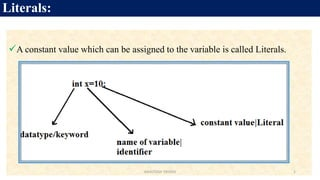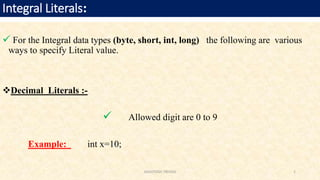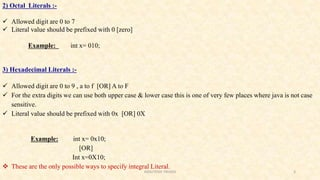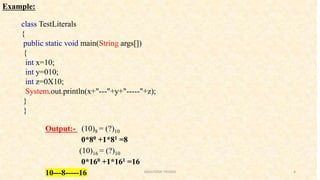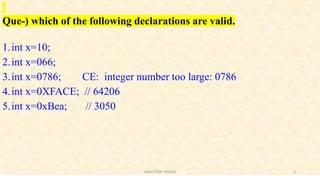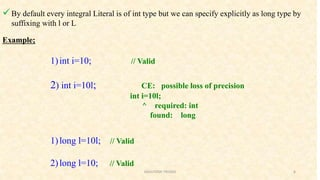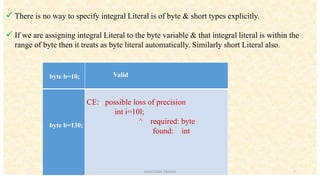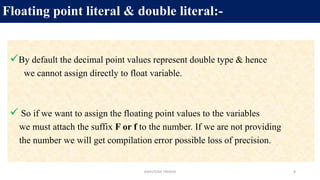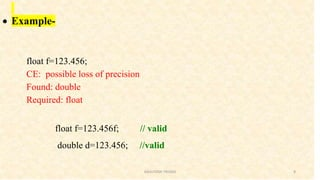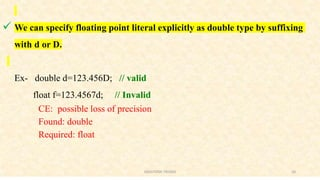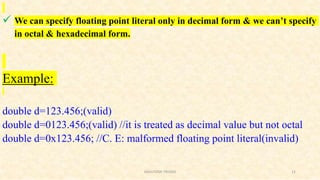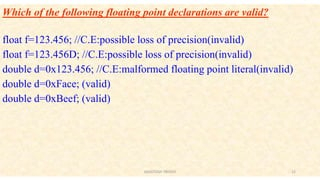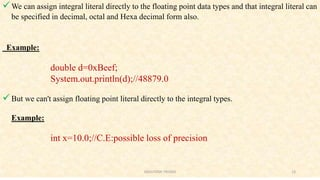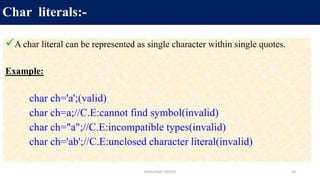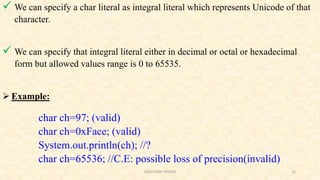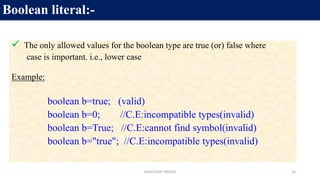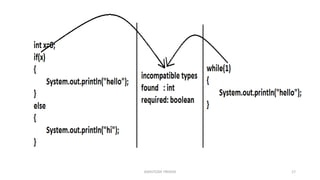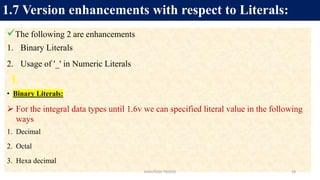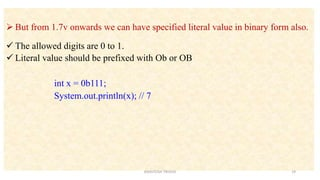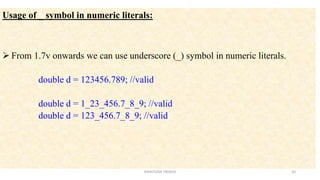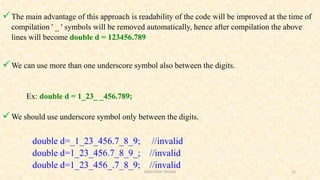1 von 21
Anzeige

### JAVA Literals

1. Literals: A constant value which can be assigned to the variable is called Literals. 1ASHUTOSH TRIVEDI
2.  For the Integral data types (byte, short, int, long) the following are various ways to specify Literal value. Decimal Literals :-  Allowed digit are 0 to 9 Example: int x=10; Integral Literals: 2ASHUTOSH TRIVEDI
3. 2) Octal Literals :-  Allowed digit are 0 to 7  Literal value should be prefixed with 0 [zero] Example: int x= 010; 3) Hexadecimal Literals :-  Allowed digit are 0 to 9 , a to f [OR] A to F  For the extra digits we can use both upper case & lower case this is one of very few places where java is not case sensitive.  Literal value should be prefixed with 0x [OR] 0X Example: int x= 0x10; [OR] Int x=0X10;  These are the only possible ways to specify integral Literal. 3ASHUTOSH TRIVEDI
4. Example: class TestLiterals { public static void main(String args[]) { int x=10; int y=010; int z=0X10; System.out.println(x+"---"+y+"-----"+z); } } Output:- (10)8 = (?)10 0*80 +1*81 =8 (10)16 = (?)10 0*160 +1*161 =16 10---8-----16 4ASHUTOSH TRIVEDI
5. Que-) which of the following declarations are valid. 1.int x=10; 2.int x=066; 3.int x=0786; CE: integer number too large: 0786 4.int x=0XFACE; // 64206 5.int x=0xBea; // 3050 5ASHUTOSH TRIVEDI
6. By default every integral Literal is of int type but we can specify explicitly as long type by suffixing with l or L Example; 1)int i=10; // Valid 2) int i=10l; CE: possible loss of precision int i=10l; ^ required: int found: long 1)long l=10l; // Valid 2)long l=10; // Valid 6ASHUTOSH TRIVEDI
7.  There is no way to specify integral Literal is of byte & short types explicitly.  If we are assigning integral Literal to the byte variable & that integral literal is within the range of byte then it treats as byte literal automatically. Similarly short Literal also. byte b=10; Valid byte b=130; CE: possible loss of precision int i=10l; ^ required: byte found: int 7ASHUTOSH TRIVEDI
8. By default the decimal point values represent double type & hence we cannot assign directly to float variable.  So if we want to assign the floating point values to the variables we must attach the suffix F or f to the number. If we are not providing the number we will get compilation error possible loss of precision. Floating point literal & double literal:- 8ASHUTOSH TRIVEDI
9.  Example- float f=123.456; CE: possible loss of precision Found: double Required: float float f=123.456f; // valid double d=123.456; //valid 9ASHUTOSH TRIVEDI
10.  We can specify floating point literal explicitly as double type by suffixing with d or D. Ex- double d=123.456D; // valid float f=123.4567d; // Invalid CE: possible loss of precision Found: double Required: float 10ASHUTOSH TRIVEDI
11.  We can specify floating point literal only in decimal form & we can’t specify in octal & hexadecimal form. Example: double d=123.456;(valid) double d=0123.456;(valid) //it is treated as decimal value but not octal double d=0x123.456; //C. E: malformed floating point literal(invalid) 11ASHUTOSH TRIVEDI
12. Which of the following floating point declarations are valid? float f=123.456; //C.E:possible loss of precision(invalid) float f=123.456D; //C.E:possible loss of precision(invalid) double d=0x123.456; //C.E:malformed floating point literal(invalid) double d=0xFace; (valid) double d=0xBeef; (valid) 12ASHUTOSH TRIVEDI
13. We can assign integral literal directly to the floating point data types and that integral literal can be specified in decimal, octal and Hexa decimal form also. Example: double d=0xBeef; System.out.println(d);//48879.0 But we can't assign floating point literal directly to the integral types. Example: int x=10.0;//C.E:possible loss of precision 13ASHUTOSH TRIVEDI
14. A char literal can be represented as single character within single quotes. Example: char ch='a';(valid) char ch=a;//C.E:cannot find symbol(invalid) char ch="a";//C.E:incompatible types(invalid) char ch='ab';//C.E:unclosed character literal(invalid) Char literals:- 14ASHUTOSH TRIVEDI
15.  We can specify a char literal as integral literal which represents Unicode of that character.  We can specify that integral literal either in decimal or octal or hexadecimal form but allowed values range is 0 to 65535.  Example: char ch=97; (valid) char ch=0xFace; (valid) System.out.println(ch); //? char ch=65536; //C.E: possible loss of precision(invalid) 15ASHUTOSH TRIVEDI
16.  The only allowed values for the boolean type are true (or) false where case is important. i.e., lower case Example: boolean b=true; (valid) boolean b=0; //C.E:incompatible types(invalid) boolean b=True; //C.E:cannot find symbol(invalid) boolean b="true"; //C.E:incompatible types(invalid) Boolean literal:- 16ASHUTOSH TRIVEDI
17. 17ASHUTOSH TRIVEDI
18. The following 2 are enhancements 1. Binary Literals 2. Usage of '_' in Numeric Literals • Binary Literals:  For the integral data types until 1.6v we can specified literal value in the following ways 1. Decimal 2. Octal 3. Hexa decimal 1.7 Version enhancements with respect to Literals: 18ASHUTOSH TRIVEDI
19.  But from 1.7v onwards we can have specified literal value in binary form also.  The allowed digits are 0 to 1.  Literal value should be prefixed with Ob or OB int x = 0b111; System.out.println(x); // 7 19ASHUTOSH TRIVEDI
20. Usage of _ symbol in numeric literals:  From 1.7v onwards we can use underscore (_) symbol in numeric literals. double d = 123456.789; //valid double d = 1_23_456.7_8_9; //valid double d = 123_456.7_8_9; //valid 20ASHUTOSH TRIVEDI
21. The main advantage of this approach is readability of the code will be improved at the time of compilation ' _ ' symbols will be removed automatically, hence after compilation the above lines will become double d = 123456.789 We can use more than one underscore symbol also between the digits. Ex: double d = 1_23_ _456.789; We should use underscore symbol only between the digits. double d=_1_23_456.7_8_9; //invalid double d=1_23_456.7_8_9_; //invalid double d=1_23_456_.7_8_9; //invalid 21ASHUTOSH TRIVEDI
Anzeige